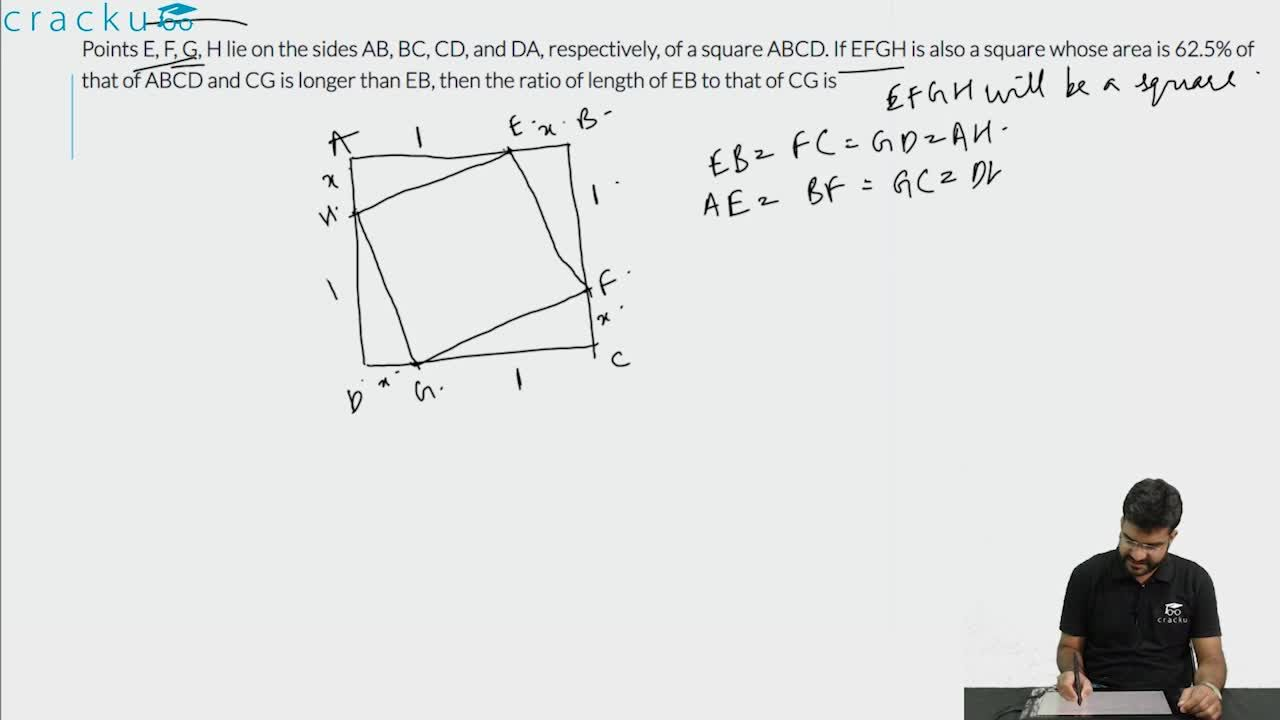Question 71

# Points E, F, G, H lie on the sides AB, BC, CD, and DA, respectively, of a square ABCD. If EFGH is also a square whose area is 62.5% of that of ABCD and CG is longer than EB, then the ratio of length of EB to that of CG is

Solution

It is given that EFGH is also a square whose area is 62.5% of that of ABCD. Let us assume that E divides AB in x : 1. Because of symmetry we can se that points F, G and H divide BC, CD and DA in x : 1.

Let us assume that 'x+1' is the length of side of square ABCD.

Area of square ABCD = $$(x+1)^2$$ sq. units.

Therefore, area of square EFGH = $$\dfrac{62.5}{100}*(x+1)^2$$ = $$\dfrac{5(x+1)^2}{8}$$ ... (1)

In right angle triangle EBF,

$$EF^2 = EB^2 + BF^2$$

$$\Rightarrow$$ $$EF = \sqrt{1^2+x^2}$$

Therefore, the area of square EFGH = $$EF^2$$ = $$x^2+1$$ ... (2)

By equating (1) and (2),

$$x^2+1 = \dfrac{5(x+1)^2}{8}$$

$$\Rightarrow$$ $$8x^2+8 = 5x^2+10x+5$$

$$\Rightarrow$$ $$3x^2-10x+3 = 0$$

$$\Rightarrow$$ $$(x - 3)(3x - 1) = 0$$

$$\Rightarrow$$ $$x = 3$$ or $$1/3$$

The ratio of length of EB to that of CG = 1 : x

EB : CG = 1 : 3 or 3 : 1. Hence, option D is the correct answer.

### View Video Solution